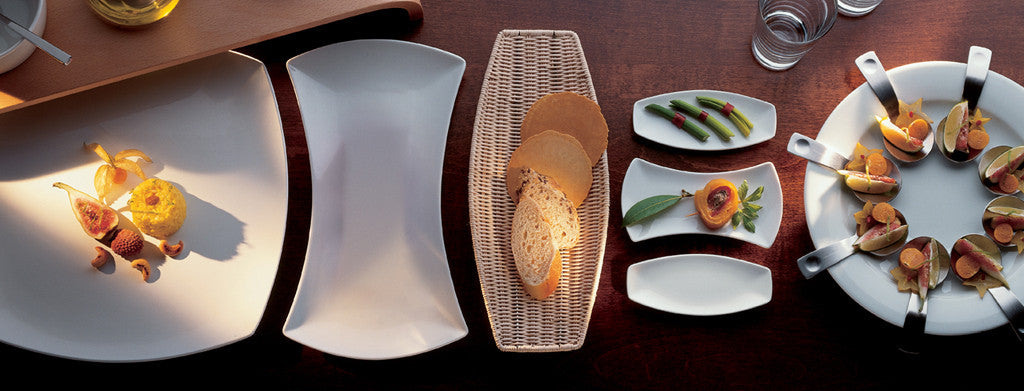Shopping Cart

### Refine

View all

#### Price# Crockery£8.17 each (ex VAT)£6.30 each (ex VAT)£5.38 each (ex VAT)£7.08 each (ex VAT)£2.39 each (ex VAT)£4.02 each (ex VAT)£2.39 each (ex VAT)£4.63 each (ex VAT)£4.02 each (ex VAT)£3.26 each (ex VAT)£7.62 each (ex VAT)£3.45 each (ex VAT)£5.93 each (ex VAT)£13.56 each (ex VAT)£13.56 each (ex VAT)£8.83 each (ex VAT)£33.57 each (ex VAT)£4.93 each (ex VAT)£2.06 each (ex VAT)£4.46 each (ex VAT)£3.18 each (ex VAT)£3.04 each (ex VAT)£17.74 each (ex VAT)£7.08 each (ex VAT)£4.90 each (ex VAT)£6.48 each (ex VAT)£6.02 each (ex VAT)£4.90 each (ex VAT)£6.48 each (ex VAT)£5.80 each (ex VAT)£4.24 each (ex VAT)£40.02 each (ex VAT)£23.99 each (ex VAT)£21.86 each (ex VAT)£7.37 each (ex VAT)£9.37 each (ex VAT)£3.97 each (ex VAT)£6.39 each (ex VAT)£23.96 each (ex VAT)£2.39 each (ex VAT)£4.63 each (ex VAT)£4.02 each (ex VAT)£2.39 each (ex VAT)£4.63 each (ex VAT)£4.02 each (ex VAT)£4.63 each (ex VAT)£2.39 each (ex VAT)£4.63 each (ex VAT)